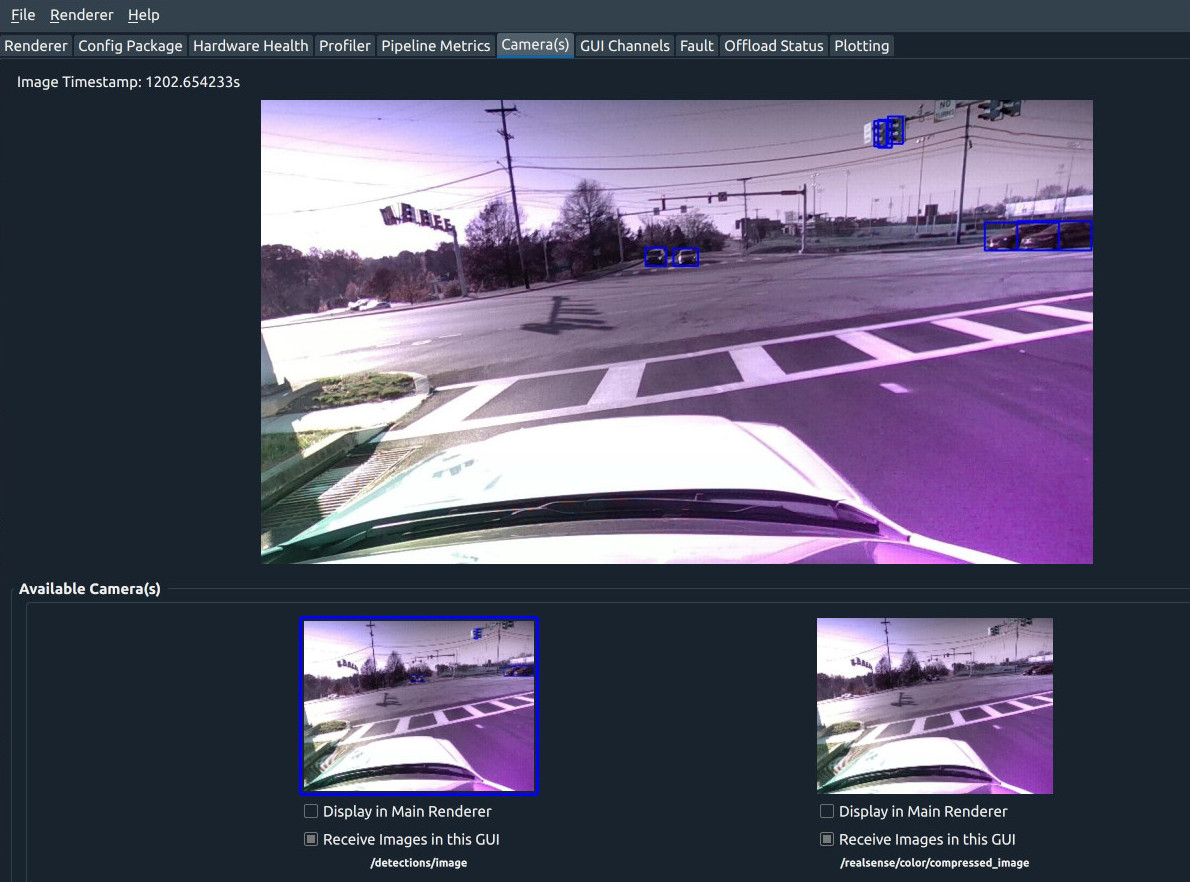# Part 5 (Running the Model)

We’ll now add some code to actually run the model and overlay the detections on the image.

And in your python `ImageDetectorStage` method, let’s add to the `handle_image` method:

``````def handle_image(self, image):
# convert image into opencv -- it's encoded as JPEG right now because that's faster
# for comms back/forth to the pipeline

# Make a copy of the image before conversion, for the overlay
original_image = cv_image.copy()

# Convert image to RGB order (we know its BGR from our log)
cv_image = cv2.cvtColor(cv_image, cv2.COLOR_BGR2RGB)

# Transpose it now for the model
cv_image = cv_image.transpose((2, 0, 1))

# Add in a batch dimension and scale pixel intensities to range [0, 1]
cv_image = np.expand_dims(cv_image, axis=0)
cv_image = cv_image / 255.0
tensor_image = torch.FloatTensor(cv_image)

# Send it to the device, and then run detection on it
device_image = tensor_image.to(self.device)
detections = self.model(device_image)

# Let's now overlay rectangles for all of the targets that we detected.
for index in range(0, len(detections['boxes'])):
# If confidence is low, ignore it
confidence = detections['scores'][index]

if confidence < 0.6:
continue

# Compute the box of where we think this object is
box = detections['boxes'][index].detach().cpu().numpy()
(left, top, right, bottom) = box.astype(int)

# Draw the bounding box on the image
cv2.rectangle(original_image, (left, top), (right, bottom), (255, 0, 0), 2)

# Now let's publish a new image out so we can see it in the gui
# we encode it as jpeg because (sigh) the Python websocket implementation
# is slow -- this will be addressed in future Ark releases.
output = Image()
output.width = image.width
output.height = image.height
output.data_format = ImageDataFormat.Jpeg.value
output.sequence_number = image.sequence_number
output.capture_time_ns = image.capture_time_ns
output.data = cv2.imencode('.jpg', original_image).tobytes()

self.image_publisher.push(output)
``````

At this point, if you run the pipeline and attach a GUI, you should see both the original camera image and the image with detections in the ‘Camera Plugin’.

An example image might look like:At this point you can do things like display the label class, add unique colors, or other fun things that are easy to do in the Python ecosystem.